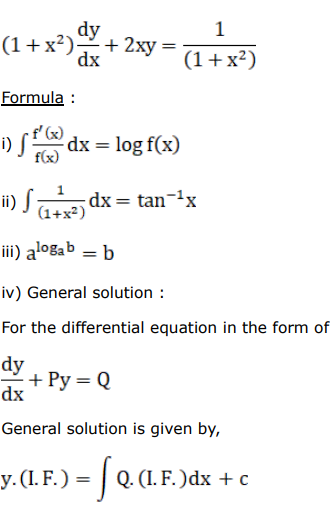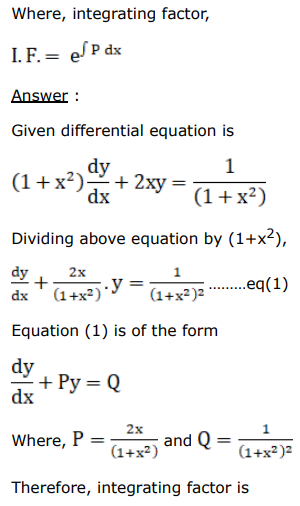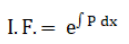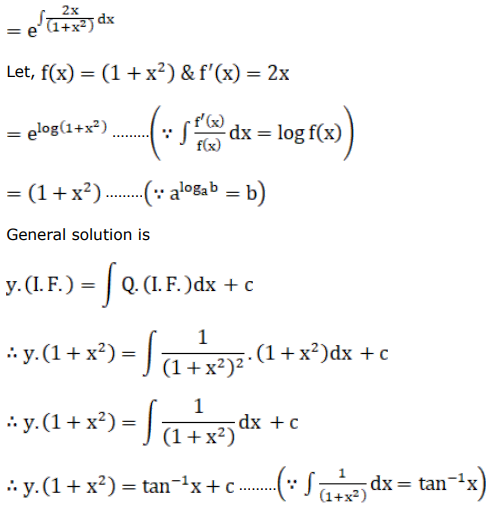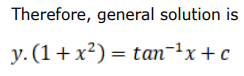# Find the general solution for each of the following differential equations.

Question:

Find the general solution for each of the following differential equations.

$\left(1+x^{2}\right) \frac{d y}{d x}+2 x y=\frac{1}{\left(1+x^{2}\right)}$

Solution:

Given Differential Equation :